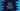# Java 8 Stream min and max method examples## Introduction :

In this tutorial, we will learn how to use min and max methods of Java 8 Stream to find the minimum and maximum element in a list. For both ’max’ and ’min’, we will pass one ’Comparator’ using which each item will be compared to each other to find the minimum or maximum values.

The syntax of ’min’ and ’max’ method is as below :

``````Optional min(Comparator<? super T> comparator)

Optional max(Comparator<? super T> comparator)``````
• It will return the maximum or minimum element of the calling stream according to the provided Comparator.
• It returns one ’Optional’ value
• If the stream is empty, it will return an empty Optional
• It will throw ‘NullPointerException’ if the minimum or maximum element is null.

Below, we will check how to find the minimum and maximum values using or without using Stream.

### Find the minimum and maximum values in a list without using Stream :

1. To find the minimum and maximum values in a list, first take the inputs from the user.
2. Create two variables ’max’ and ’min’ to store the maximum and minimum value in the list. Assign both the first number of the list.
3. Now iterate through the list one by one.
4. Check for each element: if the element is greater than current max value, set it as max. Or if it is less than current min value, set it as min.
5. After the loop is completed, print out the ’max’ and ’min’ variables.

### Java Program :

``````import java.util.ArrayList;
import java.util.Scanner;

public class Main {

/**
* Utility function for System.out.println
*
* @param message : string to print
*/
private static void println(String message) {
System.out.println(message);
}

/**
* Utility function for System.out.print
*
* @param message : string to print
*/
private static void print(String message) {
System.out.print(message);
}

/**
* main method
*
* @throws java.lang.Exception
*/
public static void main(String[] args) throws java.lang.Exception {
Scanner scanner = new Scanner(System.in);
println("How many numbers you want to add to the list : ");

//read total count entered by the user
int totalCount = scanner.nextInt();

//create one arraylist to store the numbers
ArrayList numberList = new ArrayList();

//get the inputs from the user using a 'for' loop
for (int i = 0; i < totalCount; i++) {
print("Enter number " + (i + 1) + " : ");
int number = scanner.nextInt();

}

//create two variables to store minimum and maximum values
int max = numberList.get(0);
int min = numberList.get(0);

//iterate through the arraylist and update max and min values
for (int i = 0; i < numberList.size(); i++) { //update the value of max if any number is more than it if (numberList.get(i) > max) {
max = numberList.get(i);
}

//update the value of min if any number is less than it
if (numberList.get(i) < min) {
min = numberList.get(i);
}
}

//print the min and max values
println("Minimum value in the list : " + min);
println("Maximum value in the list : " + max);

}

}``````

### Sample Output :

``````How many numbers you want to add to the list :
7

Enter number 1 : 3
Enter number 2 : 1
Enter number 3 : 98
Enter number 4 : 76
Enter number 5 : 43
Enter number 6 : 32
Enter number 7 : 65
Minimum value in the list : 1
Maximum value in the list : 98``````

Awesome. isn’t it? Now, let’s check how to achieve the same result using stream :

### Find the minimum and maximum values in a list using Stream :

To find the maximum and minimum values using stream, we will use the ’max()’ and ’min()’ methods. Let’s take a look at the program :

``````import java.util.ArrayList;
import java.util.Scanner;

public class Main {

/**
* Utility function for System.out.println
*
* @param message : string to print
*/
private static void println(String message) {
System.out.println(message);
}

/**
* Utility function for System.out.print
*
* @param message : string to print
*/
private static void print(String message) {
System.out.print(message);
}

/**
* main method
*
* @throws java.lang.Exception
*/
public static void main(String[] args) throws java.lang.Exception {
Scanner scanner = new Scanner(System.in);
println("How many numbers you want to add to the list : ");

//read total count entered by the user
int totalCount = scanner.nextInt();

//create one arraylist to store the numbers
ArrayList numberList = new ArrayList();

//get the inputs from the user using a 'for' loop
for (int i = 0; i < totalCount; i++) {
print("Enter number " + (i + 1) + " : ");
int number = scanner.nextInt();

}

int max = numberList.stream().max(Integer::compare).get();
int min = numberList.stream().min(Integer::compare).get();

//print the min and max values
println("Minimum value in the list : " + min);
println("Maximum value in the list : " + max);

}

}``````

Using only one line, we got the maximum or minimum value of the list.

• First call the ’list.stream()’ to get a ’Stream
• Then call ’max(Integer::compare)’ or ’min(Integer::compare)’ to get the maximum or minimum optional value of the list.
• And finally call ’.get()’ on it to get the integer value of the optional max or min.

### Sample Output :

``````How many numbers you want to add to the list :
7

Enter number 1 : 12
Enter number 2 : 543
Enter number 3 : 312
Enter number 4 : 21
Enter number 5 : 98
Enter number 6 : 32
Enter number 7 : 34
Minimum value in the list : 12
Maximum value in the list : 543``````